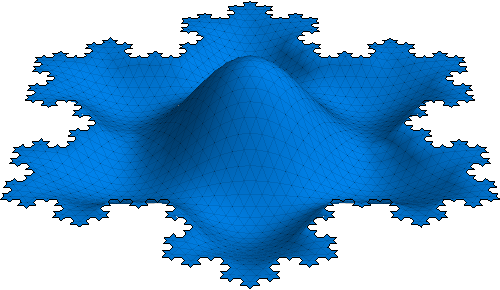# FAQ overflow

#### QUESTION

How can I call MATLAB functions directly from Mathematica and transfer data/variables between the two systems?

2014-04-12 NOTICE: The recently released MATLAB R2014a contains a bug which partially breaks MATLink (it's not possible to MGet logical type variables, which in turn may have further consequences). If you depend on MATLink, please consider keeping MATLAB R2013b until the problem is sorted out. Due to the nature of the problem there is no quick workaround and a non-trivial fix on the MATLink side would take a while.

Note: If you're using this package, please let us know how! Understanding how people use it helps us improve it in the right areas.

There is a new cross platform package for this, called MATLink. It allows calling MATLAB functions seamlessly, directly from Mathematica, as well as transferring data between the two systems. See below for a small tutorial:Disclosure: I am one of the developers of MATLink.

###### Installation

SystemOpen@FileNameJoin[{\$UserBaseDirectory, "Applications"}]



Needs["MATLink"]



and launch MATLAB using

OpenMATLAB[]



This will launch a new MATLAB process in the background that Mathematica can communicate with.

To evaluate arbitrary MATLAB commands, use MEvaluate. The output will be returned as a string.

MEvaluate["magic(4)"]

(* ==>
ans =

16     2     3    13
5    11    10     8
9     7     6    12
4    14    15     1
*)



To transfer data to MATLAB, use MSet:

MSet["x", Range]
MEvaluate["x"]
(* ==>
x =

1     2     3     4     5     6     7     8     9    10
*)



To transfer data back, use MGet:

MGet["x"]

(* ==> {1., 2., 3., 4., 5., 6., 7., 8., 9., 10.} *)



Many data types are supported, including sparse arrays, structs and cells.

MATLAB functions can be wrapped using MFunction and called directly from Mathematica:

eig = MFunction["eig"]
eig[{{1, 2}, {3, 1}}]

(* ==> {{3.44949}, {-1.44949}} *)



See the docs for more advanced usage and other functionality.

###### Simple examples

Plot the membrane from MATLAB's logo in Mathematica and manipulate the vibration modes:

Manipulate[
ListPlot3D@MFunction["membrane"][k],
{k, 1, 12, 1}
]A bucky ball straight from MATLAB:

AdjacencyGraph@Round@MFunction["bucky"][]Show Mathematica data in a zoomable MATLAB figure window:

mlf = LibraryFunctionLoad["demo_numerical", "mandelbrot", {Complex}, Integer];
mandel = Table[mlf[x + I y], {y, -1.25, 1.25, .002}, {x, -2., 0.5, .002}];
MFunction["image", "Output" -> False][mandel]

`See the webpage for a few more complex examples.

Bugs and problems: If you find any, please do report them in email (matlink.m at gmail), on GitHub, or by commenting on this post.